# Interview Questions and Answers on Amplitude Modulation

### Q.1.   Write the expression for AM wave ?

Ans.   The standard equation for amplitude modulated (AM) wave may be expressed as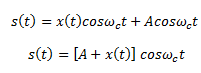### Q.2.  What is the envelope of AM wave ?

Ans.   The expression for AM wave is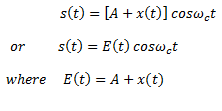E(t) is called the envelope of AM wave. This envelope consists of the baseband signal x(t). Hence, the modulating or baseband signal may be recovered from an AM wave by detecting the envelope.

### Q.3.  What is BW for AM wave ?

Ans.  The difference between these two extreme frequencies is equal to the bandwidth of the AM wave.

### Q.4.  What are the frequency components in an AM wave?

Ans.  The AM signal has three frequency components as follows :

(i)  Career frequency ωhaving amplitude A

(ii)  Upper sideband ( ω+ ωm) having amplitude ma.A/2

(iii) Lower sideband ( ωc – ωm)   having amplitude ma.A/2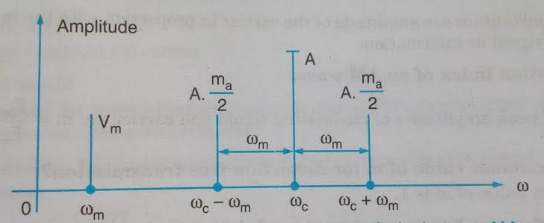Figure.1 . Single-sided frequency spectrum of single-tone AM wave

With the help of these frequency components, we can plot the frequency-spectrum of single-tone amplitude modulated (AM) wave. Figure.1 shows the one-sided frequency spectrum of single-tone AM wave.

### Q.5.  Define transmission efficiency in AM wave?

Ans.   In AM wave, the amount of useful message power Pmay be expressed by a term known as transmission efficiency η .

### Q.6.  What is demodulation ?

Ans.   The process of extracting a modulating or baseband signal from the modulated signal is called demodulation or detection. In other words, demodulation or detection is the process by which the message is recovered from the modulated signal at receiver . The device used for demodulation or detection are called demodulators or detectors. for amplitude modulation, detectors or demodulators are catagorized as :

(i)   Square-Law detectors

(ii)  Envelope detectors

### Q.7.  How can you obtain a DSB-SC signal ?

Ans.   A DSB-SC signal is obtained by simply multiplying modulating signal x(t) with carrier signal cosωct. This is achieved by a product modulator. The block diagram of a product modulator is shown in figure.2 .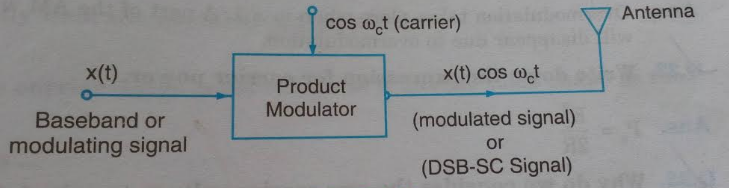Figure.2. Block diagram of Product modulator

### Q.8.  What is the BW of DSB-SC signal ?

Ans.   Bandwidth,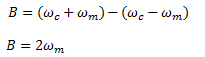It is obvious that the bandwidth of DSB-SC modulation is same as that of general AM wave .

### Q.9.  What are the demodulation methods for DSB-SC signal ?

Ans.   The DSB-SC signal may be demodulated by following two methods :

(i)  Synchronous detection method

(ii) Using envelope detector after carrier re-insertion

### Q.10.  What are the generating methods for SSB-SC signal ?

Ans.   SSB-SC signals may be generated by two methods as under :

(i)  Frequency discrimination method or Filter method

(ii) Phase discrimination method or Phase-shift method

### Q.11.  What do you mean by multiplexing ?

Ans.   Multiplexing is a technique in which several message signals are combined into composite signal for transmission over a common channel . In order to transmit a number of these signals over the same channel, the signals must be kept apart so that they do not interfere with each other, and hence they can be separated easily at the receiver end.

### Q.12.  How is the information transmitted using AM ?

Ans.   By varying the instantaneous amplitude of the carrier in proportion with the instantaneous amplitude of the message signal or information.

### Q.13.  Define modulation index of an AM wave.

Ans.   It is the ratio of peak amplitudes of modulating signal and carrier i.e.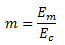### Q.14.   What is the maximum value of m for distortion free transmission ?

Ans.   Ideally maximum value of m is 1 .

### Q.15.   What is the difference between percent modulation and modulation index ?

Ans.   Percent modulation is modulation index expressed as percentage .

### Q.17.   What all frequency components are present in AM wave spectrum ?

Ans.      Carrier  fc  , Upper sideband ( fc+ f) and lower sideband ( f– f) .

### Q.18.   Which of these frequency components contain the message information ?

Ans.      Only the upper and lower sidebands.

Ans.     2 f.

### Q.20.   How do we vary the amplitude of sidebands ?

Ans.     By varying the modulation index m, if carrier amplitude is constant .

Since amplitude of each sideband = m E/2 .

### Q.21.   When does overmodulation take place and what is its effect ?

Ans.     Overmodulation takes place when m > 1 . A part of the AM wave which crosses the zero axis (X-axis) will disappear due to overmodulation .

### Q.23.   Why do we consider the rms carrier voltage to calculate the carrier power ?

Ans.     Because rms value decides the amount of power dissipated in the radiation resistance R of an antenna .

### Q.24.   Write the expression for sideband power in AM .

Ans.     Power  in each sideband = m² P/4 .

### Q.25.   What is the maximum value of sideband power in AM ?

Ans.     Maximum power in each sideband = P/4 ( at m=1 ) .

### Q.26.  What is the maximum transmitted power in AM ?

Ans.    The maximum transmitted power Pt(max) = 1.5 Pc  (at m = 1) .

### Q.27.  What is the effect of m on the carrier power in AM ?

Ans.    No effect, Pc  remains constant independent of m .

### Q.28.  Define transmission efficiency. What is its maximum value for AM ?

Ans.    It is the ratio of transmitted power which contains information to the total transmitted power .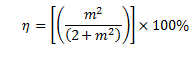Its maximum value is 33.33% at m = 1.

### Q.29.  What is the other name of AM ?

Ans.    DSB-FC i.e. Double SideBand Full Carrier .

### Q.30.  Where is the AM used ?

Ans.    AM is exclusively used for radio broadcasting application . For axample Pune A station uses AM for broadcasting its programs.

### Q.31.  Write the expression for total modulation index for simultaneous modulation by “n” modulating signals .

Ans.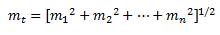### Q.32.  Why is an AM signal get severely affected due to noise?

Ans.   Because the information is contained in the amplitude variations of an AM wave, and the noise modifies the amplitude of the AM signal. Thus due to noise the information will be contaminated.

### Q.33.  For a trapezoidal pattern displayed on the CRO screen, what is the expression for the modulation index ?

Ans.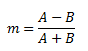### Q.34.  For an AM signal represented by eM  = 10 ( 1+ 0.4 COS 6280 t ) cos (6.28 × 106t). What are the value of modulation index and carrier frequency ?

Ans.   m = 0.4, f= 1× 10Hz i.e. 1 MHz .

### Q.35.  During modulation, the information signal is called as _____ and high frequency signal is called as  ______ .

Ans.   Modulating signal and carrier

Ans.   Higher

### Q.37.   The outline of the peak of the carrier signal is called ____ and it has the same shape as the _______.

Ans.   Envelope , Modulating signal

Ans.   Constant

### Q.39.  The amplitude modulator works on the principle of

(a)   Multiplier

(c)  Subtraction

(d)  Division

Ans.   (a) Multiplier

Ans.   m × 100%

### Q.41.  Which of the following statement is most correct ?

(a) Eshould be equal to Ec

(b) Eshould be greater than Ec

(c) Eshould be less than or equal to Ec

Ans.   c

Ans.   10 V.

Ans.   1.

### Q.44.  A signal whose amplitude is displayed with respect to time is called as ______.

Ans.   Time domain display.

### Q.45.   A signal whose amplitude is displayed with respect to frequency is called as _____ .

Ans.   Frequency domain display.

### Q.46.   Why should be carrier be a pure sine wave and should not contain any harmonics ?

Ans.   Because the harmonics of the carrier will be treated as “stations” by a receiver. So they will interfere with the already existing stations at or near these frequencies .

### Q.47.   For a carrier signal of 1 MHz and modulating signal of 2 kHz, what is the frequency range occupied by the AM signal ?

Ans.   998 kHz to 1002 kHz .

### Q.48.   In AM, the modulating frequency should always be :

(a) Greater than fc

(b) Lower than fc

(c) Equal to fc

Ans.   (b)

### Q.49.   Choose the correct statement in AM.

(a)  Sideband power is always constant

(b) Total transmitted power is constant

(c) Carrier power is constant

(d) Bandwidth is infinite .

Ans.   (c)

### Q.50.   For an AM wave represented by 10 [ 1+ 0.6 cos (2π ×1000 t )] cos ( 2π × 106 t ) the sideband frequencies are :

(a) 998 kHz and 1002 kHz

(b) 999 kHz and 1001 kHz

(c) 0.998 MHz and 1.002 MHz

(d) None of the above

Ans.   (b)

(a) 112.5 W

(b) 125 W

(c) 150 W

(d) 100 W

Ans.   (a)

(a) 150 W

(b)  200 W

(c) 50 W

(d) 100 W

Ans.   (d)

### Q.53. The total transmitted power is proportional to square of :

(a) Peak antenna current

(b) RMS antenna current

(c) Average antenna current

(d) Peak to peak antenna current

Ans.   (c)

### Q.54.  A carrier is amplitude modulated simultaneously by two sine waves with modulation indices of 0.3 and 0.6. the effective modulation index is :

(a) 0.9

(b) 0.3

(c) 0.67

(d) None of the above

Ans.   (c)

### Q.55.  The AM signal that occupies the greatest bandwidth is the one modulated by ______ .

(a) 5 kHz sine wave

(b) 2 kHz sine wave

(c) 5 kHz square wave

Ans.   (c)

### Q.57.  The total transmitted power in AM is the sum of ______ and ______ .

Ans.  Carrier  power and side band power.

Ans.  0.6324

### Q.60.  Disadvantages of AM are _____ .

Ans.

1. Larger BW requirement
2. Large power requirement

### Q.61.  State advantages of AM .

Ans.

1. Simplicity
2. Cost efficient
3. AM can travel long distance
4. Smaller BW as compared to FM